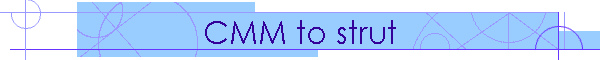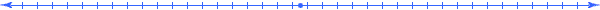Home ] What's new! ] Suspension types ] Tutorials ] Downloads ] Data files ] FAQ ] Purchase here ] Contact us ]

Converting 3D Coordinate Measuring Machine (CMM) data to SusProg3D strut dimensionsOften, vehicle manufacturers and professional car builders are able to provide coordinates for the various suspension points, usually obtained with a very sophisticated (and expensive) 3D Coordinate Measuring Machine. Usually these dimensions are "ground based".

This tutorial is intended to demonstrate the conversion of Coordinate Measuring Machine (CMM) coordinates to the SusProg3D coordinates, specifically to obtain the SusProg3D strut coordinates.

The front suspension CMM data supplied for this exercise was provided in imperial dimensions, and was in the following format.

 Front suspension - RH X Y Z Wheel centre -83.501 29.778 3.270 Axle end point -83.501 23.795 3.121 Strut rod top, chassis strut mounting -82.193 19.173 22.913 Strut rod bottom -84.492 23.945 8.500 A-arm ball joint -83.945 26.772 0.654 Steering arm balljoint -89.012 27.898 1.598 Wheel offset 0.984

The critical requirement is that the data must provide two data points on the wheel axis. Usually one will be on the wheel centreline, and the other will be on the axle end. These points will enable all the strut coordinates, wheel alignment (camber, caster and toe), and track to be determined.

You will need SusProg3D, version 4.51D (or later) to get the latest CMM to Strut tool.
This tutorial requires a registered copy of SusProg3D. It will not work with the evaluation version.

Start SusProg3D.

This tutorial will use imperial units, and a custom axis system to match the supplied data.

Go to Settings -> Settings -> Units. Select Imperial, then OK.

Just by looking at the supplied data, it can be seen that X is the longitudinal axis with +ve rearwards; Y is the lateral axis with +ve RH, and Z is the vertical axis with +ve upwards.

Go to Settings -> Settings -> Axis System.
Change the lateral axis identifier to "Y" and "+ve RH"; the vertical axis identifier to "Z" and "+ve up"; and the longitudinal axis identifier to "X" and "+ve rear". Click OK.

Check that the wheel mounting flange dimension is correctly specified.
Go to Settings -> Settings -> Wheel mounting flange.
Select Offset, then OK.Before using the CMM tool, we need to specify the basic vehicle configuration and reference data.

Select the Vehicle tab, then each of the following items in turn.

Config

For the front geometry, choose "strut". The remainder of the items can be left as-is.

Click OK.

Front Wheel

The only thing we need is the offset (0.984") and the toe reference length (17.000" to match the assumed rim diameter).

Make sure that "identical" is ticked. Click OK.

This has now completed the basic vehicle data. At this point it is probably wise to save the data.

Note that we have not entered enough data for a Geometry calculation, but only the minimum data for the CMM tool.

Go to File -> Save As.
Specify an appropriate directory and file name.

At this stage there is no graphic display, other than a small cross in the centre.Now we can enter the CMM dimensions.

Select the Tools tab.

We are working with the front suspension, and will input data for the RH side.
The first menu item should be [Front]. If it is [Rear] then click it, and change it to [Front].
The next menu item should be [LH]. If it is [RH] then click it, and change it to [LH].
Hint: If the CMM data has one side of the vehicle with +ve lateral dimensions, and the other side with -ve lateral dimensions, then always use the side that corresponds to the +ve dimensions. We chose RH because the lateral dimensions are +ve RH. If you have the lateral axis +ve LH, then choose LH here.

Select the CMM tool, and then the CMM to Strut tab.

Enter the CMM coordinate data

The spindle/wheel centre dimensions are: Y = 29.778", Z = 3.270", X =  -83.501"

The spindle reference dimensions are: Y = 23.795", Z = 3.121", X = -83.501"

The strut rod top dimensions are: Y = 19.173", Z = 22.913", X =  -82.193"

The strut rod bottom dimensions are: Y = 23.945", Z = 8.500", X = -84.492"

The wishbone balljoint dimensions are: Y = 26.772", Z = 0.654", X =  -83.945"

The steering arm balljoint dimensions are: Y = 27.898", Z = 1.598", X = -89.012"

The strut top mounting dimensions are: Y = 19.173", Z = 22.913", X =  -82.193"

Calc.

Just click the Calc.
This will do all the calculations.The first time we do the calculations, note that the tyre rolling radius is calculated as 3.271"
Although not shown in the sample data above, we have been told that the the vertical datum used by the CMM is a chassis reference point, and is 9.226" above ground. So we need to adjust all the Z dimensions by 9.226" to get to the ground plane.

If we did not know that the CMM vertical datum was not the same as ground, the calculated strut dimensions would still be accurate. What would be incorrect, is the calculated tyre rolling radius and the wheel location. We could make a reasonable guess as to the CMM vertical datum if we knew the fitted tyre size, and could estimate a rolling radius.

Since we know the CMM vertical datum, go to the CMM Setup tab.

Specify the CMM datum offset Z = 9.226". Leave the Y and X at zero.
Add a note "CMM vertical datum at chassis reference point".
Click "Calc"

Now back to the CMM to Strut tab and redo the Calculation.
The tyre rolling radius is now 12.500" which is correct.

If the wheel spindle is not on the strut rod axis (in side view) then this will be shown as “Strut rod axis to spindle offset”.
The strut dimensions are all calculated relative to a vertical strut rod axis (in side view). In this example, because the longitudinal axis is positive rearwards, the positive value indicates that the spindle is behind the strut rod axis 1.819", hence the strut rod X data points are negative (ie in front of the spindle by 1.819").

If "identical" is ticked, then the specified CMM coordinates will be calculated for the opposite side of the vehicle as well.

Hint.
Even if the vehicle is not symmetrical, it may still be quicker to assume that it is, as this will mean that the other side CMM data is set to the same is this side (with the sign change for the lateral dimensions). You can then "swap sides", open a second CMM tool, and then change whichever dimensions are different, without having to reenter all of them.

Press Control+S and save the CMM data.

Vehicle

If the vehicle is symmetrical, then tick the “identical” box, and then this will update both LH and RH struts.
If the vehicle is not symmetrical, then leave this box unticked. You will then need to switch sides, open a second copy of the tool, and input the CMM data for the other side. Remember that if you are doing the side which is the negative lateral axis, then all the lateral dimensions must be entered as negative.

Save the CMM data, use Control+S as a shortcut for File | Save.

If you want to use these calculated values to update the vehicle data, then press “Vehicle”.
The strut dimensions will be carried over to the Geometry | Strut input dialog, the strut type will be set to “Fixed knuckle/strut A” and the steering arm location will be set to “knuckle”.
The tyre rolling radius will be carried over to the Geometry | Wheel input dialog.
The wheel alignment and location will be carried over to the Geometry | Alignment input dialog.Well, that's enough for this tutorial.

Now we have the strut dimensions, wheel and tyre dimensions, and basic setup alignment.

You can now enter the rest of the data, such as chassis mounting points, ride heights etc.

Note that the CMM data is stored in the SusProg3D data files. After doing the geometry calculation, you can look at the results, and verify that the calculated points agree with the initial CMM data.

Now, it is easy to start varying.

What if a smaller tyre is fitted?

What if the camber is changed?

All of this without having to recalculate the CMM coordinates! And that is the main advantage of having the strut coordinates in their own axis system. The freedom to move and realign.